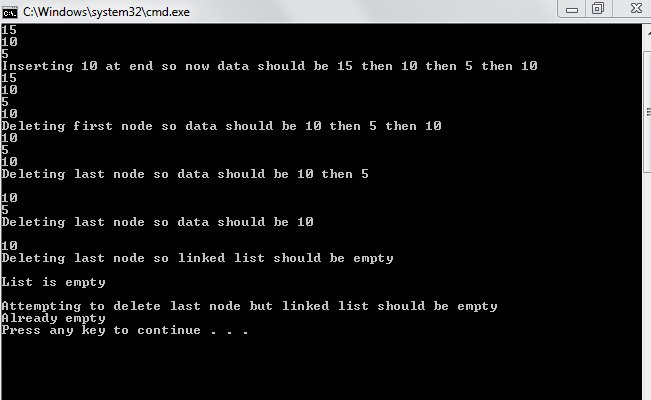# Linked List Basic Functions Part 3

In this tutorial, you will learn 1. Implementation of function for inserting a node at end. 2. Implementation of function for deleting first node. 3. Implementation of function for deleting last node. What is the code of function for insertion of a node at end?
`void InsertAtEnd(int val){    if (checkempty()){Node *nnode=new Node;nnode->data=val;nnode->next=head;head=nnode;} else{ 	Node * curr;curr=head;Node *curr1;curr1=curr->next;while(curr1!=NULL){curr=curr->next;curr1=curr1->next;}Node *nnode=new Node;nnode->data=val;curr->next=nnode;} }`
Given above is the code for inserting a node at end. First, you check if the linked list is empty. If its empty, then the code for inserting at beginning will work. If its not empty, then you make two pointers curr and curr1. curr1 will point to node next to curr. When curr1 will become null then curr will point to last node. What we do is that we simply put the address of new node in next of last node. Hence, link is made. What is the code for deleting the first node?
`void DeleteFirstNode(){	if(checkempty())	{		cout<<"Already empty";	}	else	{		Node * curr;		curr=head;		head=head->next;		delete curr;	}}`
Given above is the code for deleting first node. You first check whether the linked list is empty or not. If empty then it cannot delete any node. If its not empty, first you make a pointer and make it equal to head so that it points to first node. After that, make head equal to 2nd node and delete first node. Remember deleting a pointer means deleting the object it points to and as while inserting, we were making dynamic nodes ( As new operator was used ) so that memory must be deleted manually. What is code for deleting last node?
`void DeleteLastNode(){	if(checkempty())	{		cout<<"Already empty";	}	else if (head->next==NULL)	{		Node * curr;		curr=head;		head=head->next;		delete curr;	}	else 	{		Node *curr;		Node *curr1;		curr=head;		curr1=curr->next;		while(curr1->next!=NULL)		{			curr=curr->next;			curr1=curr1->next;		}		curr->next=NULL;		delete curr1;}}`
Given above is the code for deleting the last node of linked list. First we check whether the list is empty or not. If empty, you cannot delete a node. Then, we check whether the linked list has just one node. If the next of first element (head) is NULL then there is just one node. If there is one node, method of deleting a single node works. If more than one nodes, we make two pointers curr and curr1. curr1 pointing to element next to curr and we stop curr1 at last node so curr points second last. Lastly, we make the next of second last element equal to null and delete last element. Note: Basic functions of insertion, deletion, traversal, constructor and check if empty are discussed. In next tutorial, copy constructor, destructor and sorting to make the linked list will be discussed. Then after that insertion and deletion at any point will be discussed along with comparison between effiviency of different functions in arrays and linked list. Output: